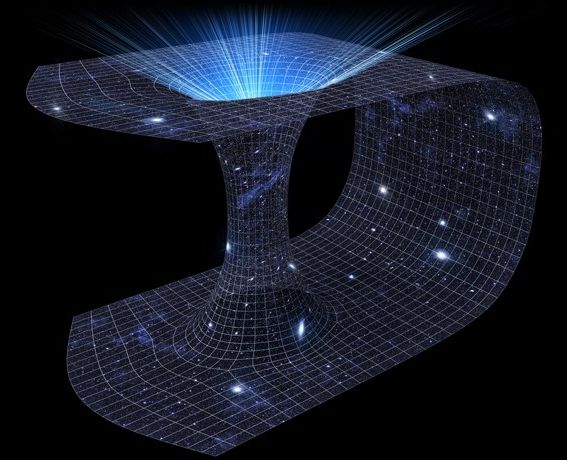## БЛОГThere’s a new equation floating around the world of physics these days that would make Einstein proud.

It’s pretty easy to remember: ER=EPR.

You might suspect that to make this equation work, P must be equal to 1. But the symbols in this equation stand not for numbers, but for names. E, you probably guessed, stands for Einstein. R and P are initials — for collaborators on two of Einstein’s most intriguing papers. Combined in this equation, these letters express a possible path to reconciling Einstein’s general relativity with quantum mechanics.

Read more